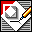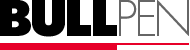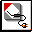Scale TablesMetric

Scale

Ratio

Scale

Ratio

Scale

Ratio

full scale

1:1

1"=10'-0"

1:120

1:1

1:1

6"=1'-0"

1:2

1"=20'-0"

1:240

1:2

1:2

3"=1'-0"

1:4

1"=25'-0"

1:300

1:2.5

1:2.5

1 1/2"=1'-0"

1:8

1"=30'-0"

1:360

1:5

1:5

1"=1'-0"

1:12

1"=40'-0"

1:480

1:10

1:10

3/4"=1'-0"

1:16

1"=50'-0"

1:600

1:12.5

1:12.5

1/2"=1'-0"

1:24

1"=60'-0"

1:720

1:20

1:20

3/8"=1'-0"

1:32

1"=80'-0"

1:960

1:50

1:50

1/4"=1'-0"

1:48

1"=100'-0"

1:1,200

1:100

1:100

3/16"=1'-0"

1:64

1"=200'-0"

1:2,400

1:200

1:200

1/8"=1'-0"

1:96

1"=500'-0"

1:6,000

1:1,000

1:1,000

3/32"=1'-0"

1:128

1:1,250

1:1,250

1/16"=1'-0"

1:192

1:2,000

1:2,000

J Richardson also notes:

 1:2,500 1:5,000 1:10,000 1:25,000 1:50,000 1:100,000 1:4,800 (1" = 400') 1:10,560 (6" = 1 mile) 1:12,000 (1" = 1,000') 1:21,120 (3" = 1 mile) 1:24,000 (1" = 2,000') 1:63,360 (1" = 1 mile) 1:126,720 (1/2" = 1 mile)Speaking of which,
in the Dept. of Whimsy:

For the ocean sailors, (who know that 1 nautical mile = 6,080.27 feet):
1:72,963.24 (1" = 1 nautical mile)

For the mountaineers out there, who know better than I do the height of the tallest mountain in North America: 1:243,840 (1" = 20,320' eg, approximately one *Denali*)

 Model Railroad scale equivalants are posted at Rick Blanchard's site: http://www.urbaneagle.com/data/RRstddims.html